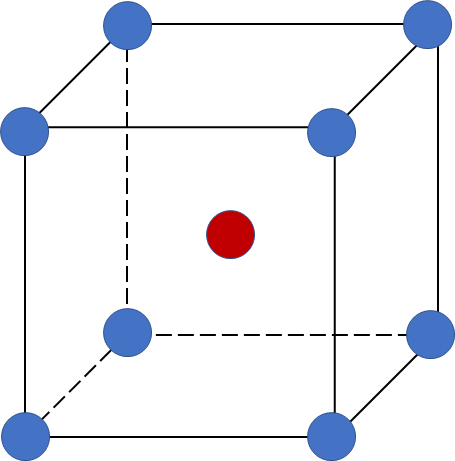# Problem: One of the most important enzymes in the world—nitrogenase, the plant protein that catalyzes nitrogen fixation—contains active clusters of iron, sulfur, and molybdenum atoms. Crystalline molybdenum (Mo) has a body-centered cubic unit cell (d of Mo = 10.28 g/cm3). Calculate the atomic radius of Mo.

###### FREE Expert Solution
80% (354 ratings)
###### FREE Expert Solution

We’re being asked to find the atomic radius of crystalline molybdenum (Mo) that has a body-centered (BCC) cubic unit cell.

A body-centered cubic (BCC) unit cell is composed of a cube with one atom at each of its corners and one atom at the center of the cubeRecall that the edge length (a) of a BCC unit cell can be calculated using the equation:

80% (354 ratings)###### Problem Details

One of the most important enzymes in the world—nitrogenase, the plant protein that catalyzes nitrogen fixation—contains active clusters of iron, sulfur, and molybdenum atoms. Crystalline molybdenum (Mo) has a body-centered cubic unit cell (d of Mo = 10.28 g/cm3). Calculate the atomic radius of Mo.

What scientific concept do you need to know in order to solve this problem?

Our tutors have indicated that to solve this problem you will need to apply the Unit Cell concept. You can view video lessons to learn Unit Cell. Or if you need more Unit Cell practice, you can also practice Unit Cell practice problems.

What is the difficulty of this problem?

Our tutors rated the difficulty ofOne of the most important enzymes in the world—nitrogenase, ...as high difficulty.

How long does this problem take to solve?

Our expert Chemistry tutor, Dasha took 13 minutes and 22 seconds to solve this problem. You can follow their steps in the video explanation above.

What professor is this problem relevant for?

Based on our data, we think this problem is relevant for Professor Oppenheim's class at University of Colorado at Colorado Springs.

What textbook is this problem found in?

Our data indicates that this problem or a close variation was asked in Chemistry: The Molecular Nature of Matter and Change - Silberberg 8th Edition. You can also practice Chemistry: The Molecular Nature of Matter and Change - Silberberg 8th Edition practice problems.